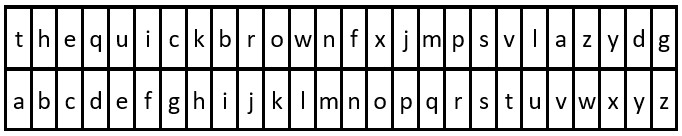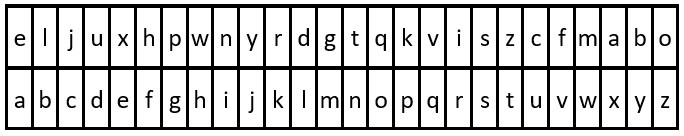# Decode the Message Leetcode Python SolutionJuly 4, 2022

Competitive programming is essential and today we are going to solve the round bounded in circle leetcode problem. This problem appeared in amazon coding interview.

The question states that:-

You are given the strings `key` and `message`, which represent a cipher key and a secret message, respectively. The steps to decode `message` are as follows:

1. Use the first appearance of all 26 lowercase English letters in `key` as the order of the substitution table.
2. Align the substitution table with the regular English alphabet.
3. Each letter in `message` is then substituted using the table.
4. Spaces `' '` are transformed to themselves.
• For example, given `key = "happy boy"` (actual key would have at least one instance of each letter in the alphabet), we have the partial substitution table of (`'h' -> 'a'`, `'a' -> 'b'`, `'p' -> 'c'`, `'y' -> 'd'`, `'b' -> 'e'`, `'o' -> 'f'`).

Return the decoded message.

Example 1:```Input: key = "the quick brown fox jumps over the lazy dog", message = "vkbs bs t suepuv"
Output: "this is a secret"
Explanation: The diagram above shows the substitution table.
It is obtained by taking the first appearance of each letter in "the quick brown fox jumps over the lazy dog".
```

Example 2:```Input: key = "eljuxhpwnyrdgtqkviszcfmabo", message = "zwx hnfx lqantp mnoeius ycgk vcnjrdb"
Output: "the five boxing wizards jump quickly"
Explanation: The diagram above shows the substitution table.
It is obtained by taking the first appearance of each letter in "eljuxhpwnyrdgtqkviszcfmabo".
```

Constraints:

• `26 <= key.length <= 2000`
• `key` consists of lowercase English letters and `' '`.
• `key` contains every letter in the English alphabet (`'a'` to `'z'`) at least once.
• `1 <= message.length <= 2000`
• `message` consists of lowercase English letters and `' '`.

Source: Leetcode

Solution:

So, Here first we will create a list of the alphabet key in order for the list index plus 97 to behave like the ASCII value of the list key value.

then we will run the loop for each index of message find its index and then plus 97 to their index find the chr accourding to the ASCII value keep concatenate with ans and return after the loop finishes.

Python solution:

``````class Solution:
def decodeMessage(self, key: str, message: str) -> str:

li = []
for i in key:
if i != ' ' and i not in li:
li.append(i)

ans = ''
for i in message:
if i==' ':
ans+=' '
else:
ans+=chr(97+li.index(i))
return ans``````

Time complexity: O(n)

Space complexity: O(26)